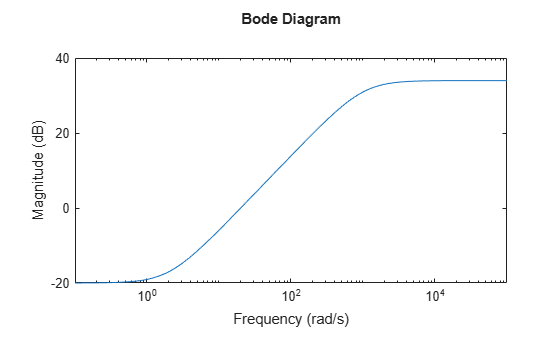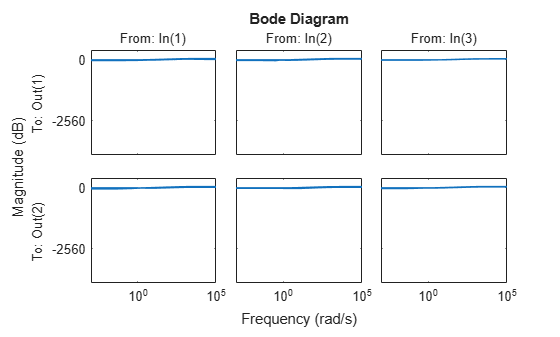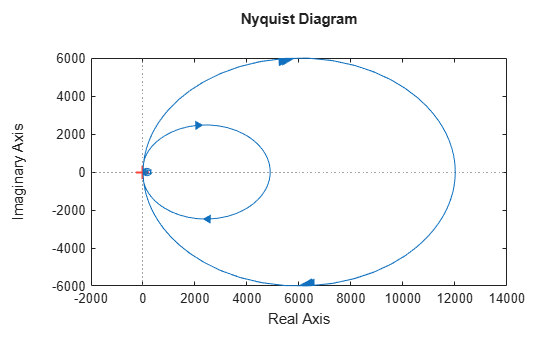# ultidyn

Create uncertain linear time-invariant object

## Syntax

```H = ultidyn('Name',iosize)
H = ultidyn('Name',iosize,'Property1',Value1,'Property2',Value2,...)
```

## Description

`H = ultidyn('Name',iosize)` creates an uncertain linear, time-invariant objects are used to represent unknown dynamic objects whose only known attributes are bounds on their frequency response. Uncertain linear, time-invariant objects have a name (the `Name` property), and an input/output size (`ioSize` property).

Trailing Property/Value pairs are allowed in the construction.

`H = ultidyn('name',iosize,'Property1',Value1,'Property2',Value2,...)`

The property `Type` is `'GainBounded'` (default) or `'PositiveReal'`, and describes in what form the knowledge about the object's frequency response is specified.

• If `Type` is `'GainBounded'`, then the knowledge is an upper bound on the magnitude (i.e., absolute value), namely `abs(H)<= Bound` at all frequencies. The matrix generalization of this is ∥`H``<= Bound`.

• If `Type` is `'PositiveReal'` then the knowledge is a lower bound on the real part, namely `Real(H) >= Bound` at all frequencies. The matrix generalization of this is `H+H' >= 2*Bound`

The property `Bound` is a real, scalar that quantifies the bound on the frequency response of the uncertain object as described above.

The property `SampleStateDimension` is a positive integer, defining the state dimension of random samples of the uncertain object when sampled with `usample`. The default value is 3.

The property `AutoSimplify` controls how expressions involving the uncertain matrix are simplified. Its default value is` 'basic'`, which means elementary methods of simplification are applied as operations are completed. Other values for `AutoSimplify` are` 'off'`, no simplification performed, and` 'full'` which applies model-reduction-like techniques to the uncertain object.

Use the property `SampleMaxFrequency` to limit the natural frequency for sampling. Randomly sampled uncertain dynamics are no faster than the specified value. The default value is `Inf` (no limit).

To model frequency-dependent uncertainty levels, multiply the `ultidyn` object by a suitable shaping filter. For example, for a `ultidyn` object `dH`, the following commands specify an uncertainty bound that increases from 0.1 at low frequencies to 10 at high frequencies.

```W = tf([1 .1],[.1 1]); dH = W*dH;```

## Examples

collapse all

Create a `ultidyn` object with internal name `'H'`, norm bounded by 7, with three inputs and two outputs.

`H = ultidyn('H',[2 3],'Bound',7) `
```H = Uncertain LTI dynamics "H" with 2 outputs, 3 inputs, and gain less than 7. ```

Typically, when you use uncertain dynamics, you apply a weighting function to emphasize the uncertain contribtion in a certain bandwidth. For instance, suppose that the behavior of your system is modestly uncertain (say 10%) at low frequencies, while the high-frequency behavior beyond 20 rad/s is not accurately modeled. Use `makeweight` to create a shaping filter that captures this behavior.

```W = makeweight(.1,20,50); bodemag(W)```Apply the weighting filter at the block outputs. Examine samples of the unmodeled dynamics.

```Hw = blkdiag(W,W)*H; bodemag(Hw)```Create a scalar `ultidyn` object with an internal name `'B'`, whose frequency response has a real part greater than 2.5.

`B = ultidyn('B',[1 1],'Type','PositiveReal','Bound',2.5)`
```B = Uncertain LTI dynamics "B" with 1 outputs, 1 inputs, and positive real bound of 2.5. ```

Change the `SampleStateDimension` to 5, and plot the Nyquist plot of 30 random samples.

```B.SampleStateDimension = 5; nyquist(usample(B,30))```## Compatibility Considerations

expand all

Behavior changed in R2020a